### MCQs on Chemical Engineering - 11

1. A hydrocarbon contains 86 % carbon, 448 ml of the hydrocarbon weighs 1.68 𝑔 of STP. Then the hydrocarbon is

(a) ALKENE
(b) ALKANE
(c) ALKYNE
(d) ARENE

2. When a solution of a metal salt is treated with ammonia solution, a white precipitate is formed which is insoluble in excess of ammonia. When same solution is treated with NaOH, a white precipitate is formed which dissolves in excess of NaOH. The cation is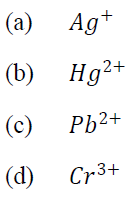3. What is the solubility product of lead iodide at 25°𝐶? Its solubility at that temperature is 0.7 𝑔/𝑙, atomic weight of Pb=207.2 and I=127.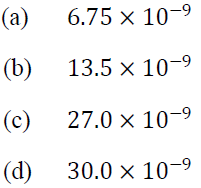4. The diameter measured under various trials for a wire using a microscope were 0.205 𝜇m, 0.209 𝜇m and 0.198 𝜇m. If the known diameter is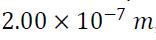, the percentage of error for an average measurement is
(a) 1.8 %
(b) 3.5 %
(c) 2.4 %
(d) 2.0 %

5. Which one of following is the correct sequence in decreasing order of the dielectric constants?
(a) Sulfuric acid > water > ethanol > decane
(b) Water >sulfuric acid > ethanol > decane
(c) Water >sulfuric acid > decane > ethanol
(d) Sulfuric acid > ethanol > water > decane

6. Which one of the following is having isotonic nuclei?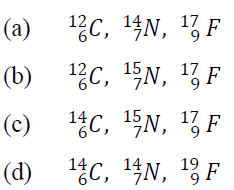7. When a stream of electrons strikes a metal target, the resulting radiation is
(a) 𝛾− Rays
(b) Electron reemission
(c) 𝑋− Rays
(d) Neutrons

8. Which of the following set of quantum numbers are possible?
1. 𝑛 = 3, 𝑙 = 0, 𝑚 = 0 and 𝑠 =+1/2
2. 𝑛 = 3, 𝑙 = 1, 𝑚 = −1 and 𝑠 = −1/2
3. 𝑛 = 2, 𝑙 = 0, 𝑚 = −1 and 𝑠 = +1/2
4. 𝑛 = 2, 𝑙 = 1, 𝑚 = 0 and 𝑠 = +1/2

(a) 1, 2 and 3
(b) 1, 3 and 4
(c) 1, 2 and 4
(d) 2, 3 and 4

9. The pH , at which the amino acid is in the dipolar form is known as:
(a) Isoelectric point
(b) Isosbestic point
(c) Mutarotation
(d) Transition point

10. The following two isomers are: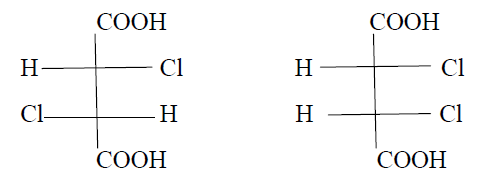(a) Enantiomers
(b) Mesomers
(c) Diastereomers
(d) Position isomers

Share:

## Featured Post

### UPSC Civil Service Preliminary Paper-1 Previous Year Solved Question Papers

Civil Service Preliminary Paper-1 Previous Year Solved Questions for the year 2019 Civil Service Preliminary Paper-1 Previous Year Solved Qu...Name

Email *

Message *